Best Engineering BTech and Management College in Kolkata West Bengal | NSEC - Laboratories of Electrical Engineering Department
::  Laboratories of Electrical Engineering Department  ::
• Basic Electrical Engg
• Electric Circuit Theory
• Electric Machine
• Electrical & Electronic Measurement
• Power System
• Control System
• Micro Processor & Microcontroller
• Power Electronics & Drives
• Basic Electrical & Electronics Engg-I Lab [ES 191] and Basic Electrical & Electronics Engg-II Lab [ES 291]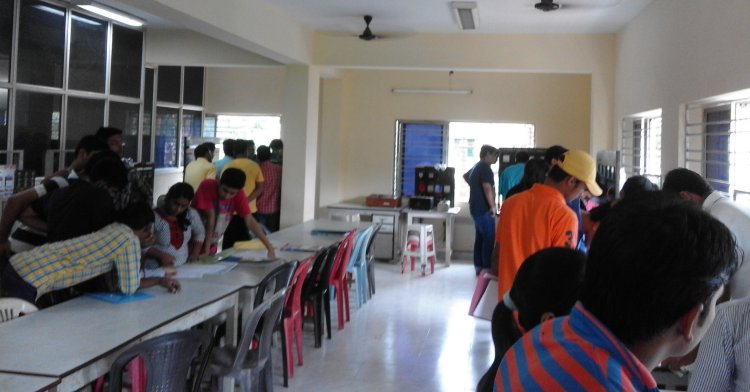Basic Electrical Engineering Laboratory-I

List of Experiments:
1. Characteristics of Fluorescent lamps
2. Characteristics of Tungsten and Carbon filament lamps
3. (a) Verification of Thevenin's theorem.
3. (b) Verification of Norton's theorems.
4. Verification of Maximum power theorem.
5. Verification of Superposition theorem
6. Study of R-L-C Series circuit
7. Study of R-L-C parallel circuit

Basic Electrical Engineering Laboratory-II

List of Experiments:
1. Calibration of ammeter and voltmeter.
2. Open circuit and Short circuit test of a single phase Transformer.
3. No load characteristics of D.C shunt Generators
4. Starting and reversing of speed of a D.C. shunt
5. Speed control of DC shunt motor.
6. Measurement of power in a three phase circuit by two wattmeter method.

• Electric Circuit Theory• Electric Machine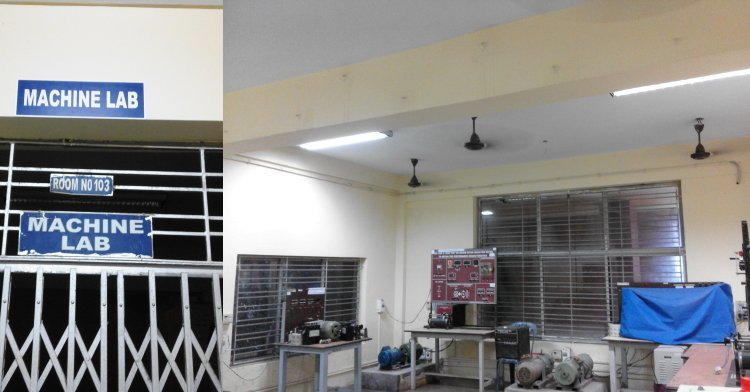• Electrical & Electronic Measurement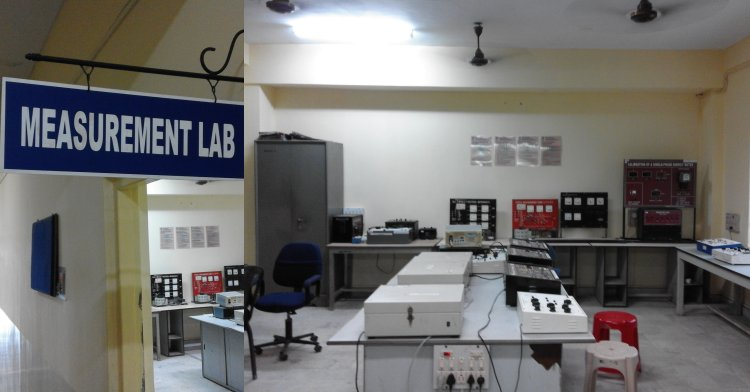• Power System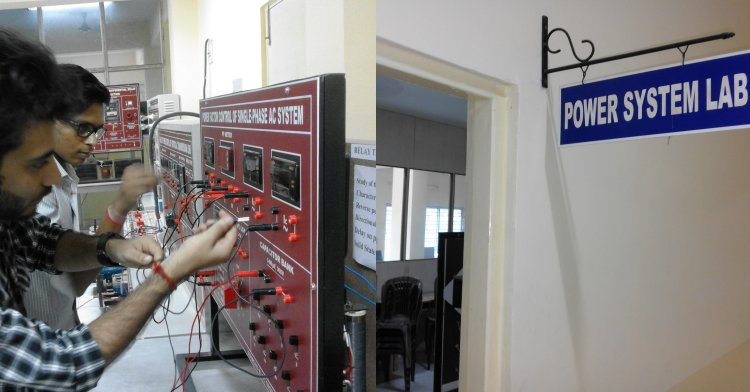• Control System• Micro Processor & Microcontroller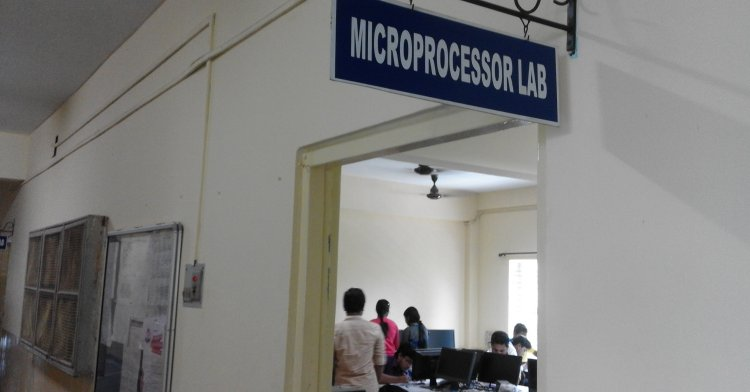• Power Electronics & Drives